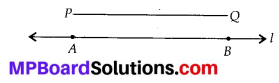# MP Board Class 6th Maths Solutions Chapter 14 Practical Geometry Ex 14.3

## MP Board Class 6th Maths Solutions Chapter 14 Practical Geometry Ex 14.3

Question 1.
Draw any line segment $$\overline{P Q}$$.Without measuring $$\overline{P Q}$$, construct a copy of $$\overline{P Q}$$.
Solution:
Steps of construction :(i) Draw $$\overline{P Q}$$whose length is not known.
(ii) Fix the compasses pointer on P and the pencil end on Q. The opening of the compasses now gives the length of $$\overline{P Q}$$
(iii) Draw any line ‘l’. Choose a point A on ‘l’. Without changing the compasses setting, place the pointer on A.
(iv) Draw an arc that cuts ‘l’ at a point, say B
Now, $$\overline{A B}$$ is a copy of $$\overline{P Q}$$.Question 2.
Given some line segment $$\overline{A B}$$, whose length you do not know, construct $$\overline{P Q}$$ such that the length of $$\overline{P Q}$$ is twice that of $$\overline{A B}$$
Solution:
Steps of construction :(i) Draw $$\overline{A B}$$ whose length is not known.
(ii) Fix the compasses pointer on A and the pencil end on The opening of the compasses now gives the length of $$\overline{A B}$$.
(iii) Draw any line ‘l’. Choose a point P on ‘l’. Without changing the compasses setting, place the pointer on
(iv) Draw an arc that cuts ‘l’ at a point R.
(v) Now place the pointer on R and without changing the compasses setting, draw another arc that cuts ‘Y at a point Q.
(vi) Thus $$\overline{P Q}$$is the required line segment whose length is twice that of AB.